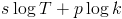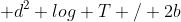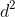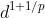### Lucene Performance

I hope here to give a brief overview of the theoretical complexity of various Lucene operations. It has been my experience that the practical performance is in line with the theoretical performance, for most practical values, but your mileage may vary. (Some of the latex requires Tex the World in order to render.)

Finding a node in a skip list takes log n operations. Since Lucene's term index is a skip list of unique terms, the query "do any documents contain term t?" will take log(number of unique terms). The query "find all documents containing t" will take log(# of unique terms) + number of matches. If your index is sharded (i.e. your index is not optimized) you will need to multiply these formulae by the number of segments, si
nce each segment has to be searched independently.

The real optimizations of Lucene come from the fact that you never search for all documents which match the query, but rather the top k. Call T the number of unique terms in your index. Say you have s sub-queries. Finding the s posting streams (one per query) takes s log T (since finding a posting stream is just finding that term in the skip list). Now suppose there are p instances of each term in your query, i.e.

Finding the top k matches can be done in p log k , so the entire search runs in, which is almost always going to be dominated by p log k. This means that 10 queries, each matching 10 documents, takes about as long as 1 query matching 100 documents (assuming k >= 100, of course). To put it another way: for standard disjunctive (OR'd) queries, the number of clauses doesn't really affect performance, except to the extent that more documents are potential matches.

This answers another common question about Lucene queries: what is the performance of "special" query types like range and prefix? The answer is that these queries add an additional penalty proportional to T, since they have to enumerate through the term index to find all terms which are in their range. However, the time actually finding documents in that range is not affected (although obviously the user will view it all as "query time").

If you've investigated Lucene performance on the indexing side of things, you know the key variable is "merge factor", or the maximum number of partitions the index can have. More partitions means faster writes and slower reads.

Say you have d documents, T unique terms and your memory can hold b documents at once. Then indexing can be done in, which is dominated by. But this is if you have only one partition. If you have p partitions, indexing can be done in (get ready for this, cause it's a doozy):

(Note that in the case where p=1, this reduces toor, which is what our original formula was dominated by). The key term there is againyou can see that going from one partition to 10 causes indexing time to fall fromto, and as the number of partitions increases, performance becomes linear (which isn't too surprising if you think about it. If p = d, then adding a document is just appending to a list, which is constant, and managing the segments file, which is linear.)

But what about the other side of things? If p = d and it's a linked list, then indexing time is linear but so is search time (which is a huge degredation from the above stated p log k). In practice, p is always much less than d (the operating system will probably throw "too many open files" if p is even in the double digits unless you're using cfs). Ignoring practical considerations though, you can essentially assume you have to run the query for each individual partition, so the number of partitions (roughly) linearly increases the time.

1. http://lucene.sourceforge.net/papers/riao97.ps
2. Lester, N., A. Moffat, and J. Zobel. “Fast on-line index construction by geometric partitioning.” In Proceedings of the 14th ACM international conference on Information and knowledge management, 783. ACM, 2005.
(note: to get the cited equation for Lester et al., substitute in the disk access formula on page 5 into the value of r for fixed p on page 6.)